# RD Sharma Solutions For Class 7 Maths Chapter 2 Fractions Exercise 2.1

Students can refer and download the RD Sharma Solutions for Class 7 Chapter 2 Fractions Exercise 2.1 from the link provided here. Our specialist tutors formulate these exercises to help you with your exam preparation to achieve good marks in Maths. This exercise can be used as a model of reference by the students to improve their conceptual knowledge and understand the different ways used to solve the problems. By practising RD Sharma Solutions for Class 7, students will be able to grasp the concepts perfectly. It also helps in boosting their confidence, which plays a crucial role in the examinations.

## Download the PDF of RD Sharma Solutions For Class 7 Maths Chapter 2 – Fractions Exercise 2.1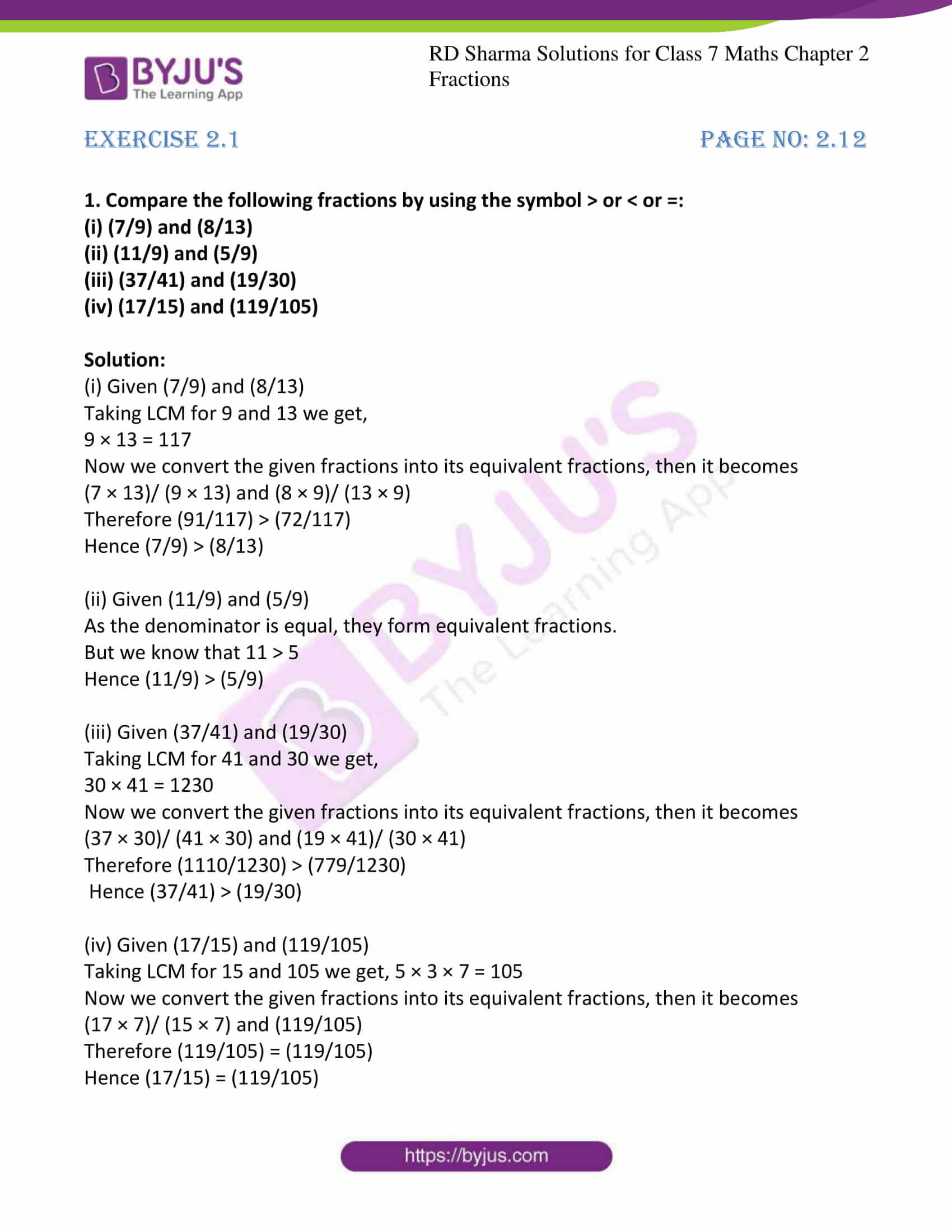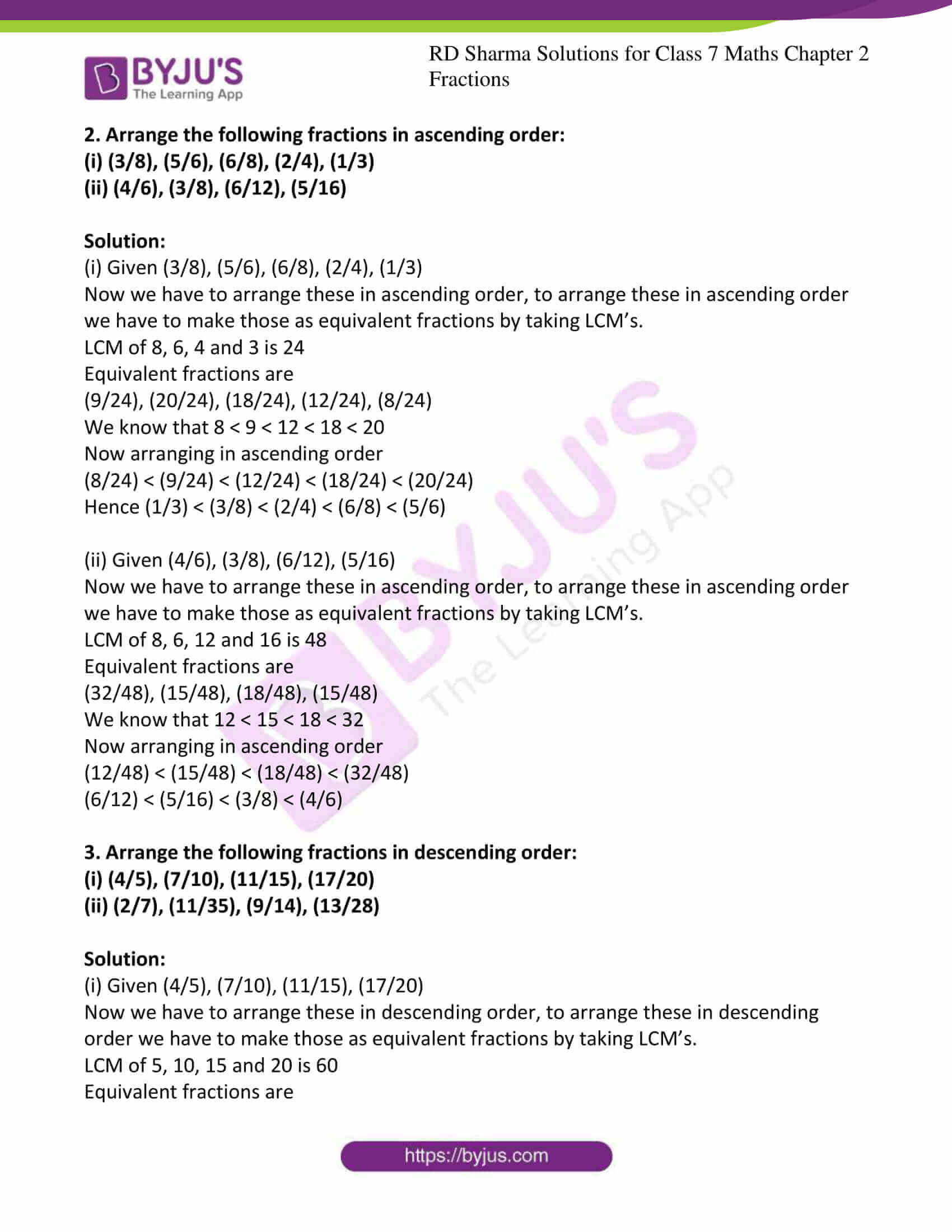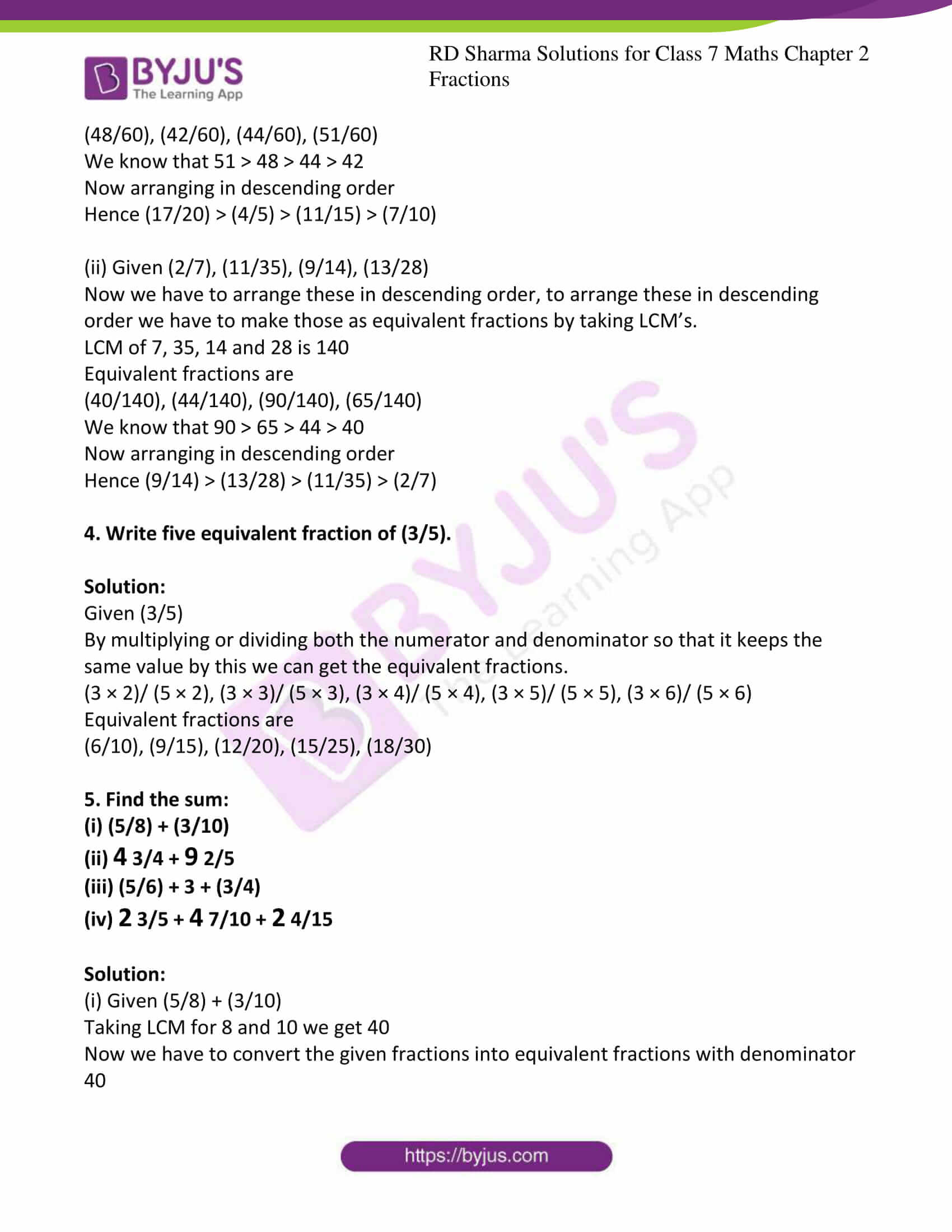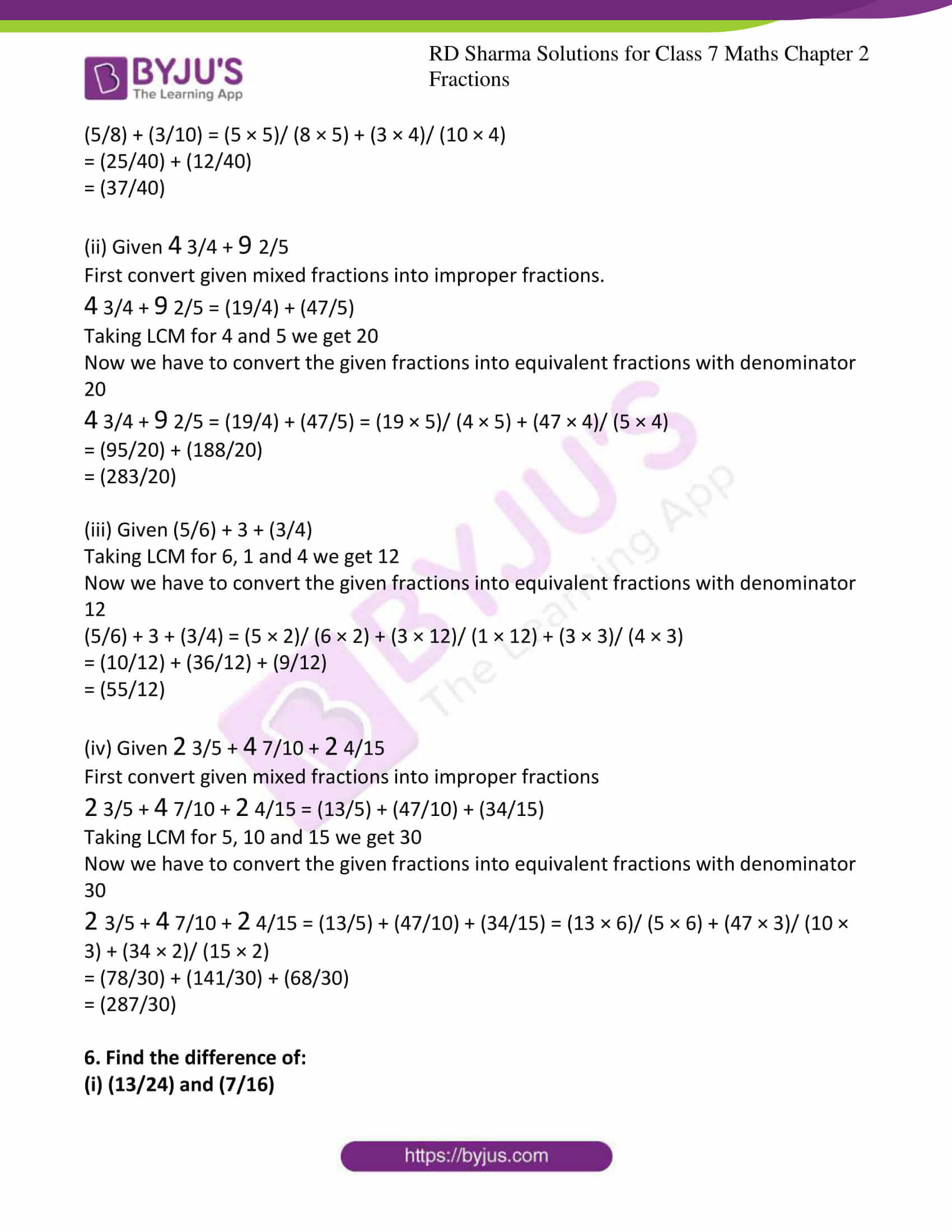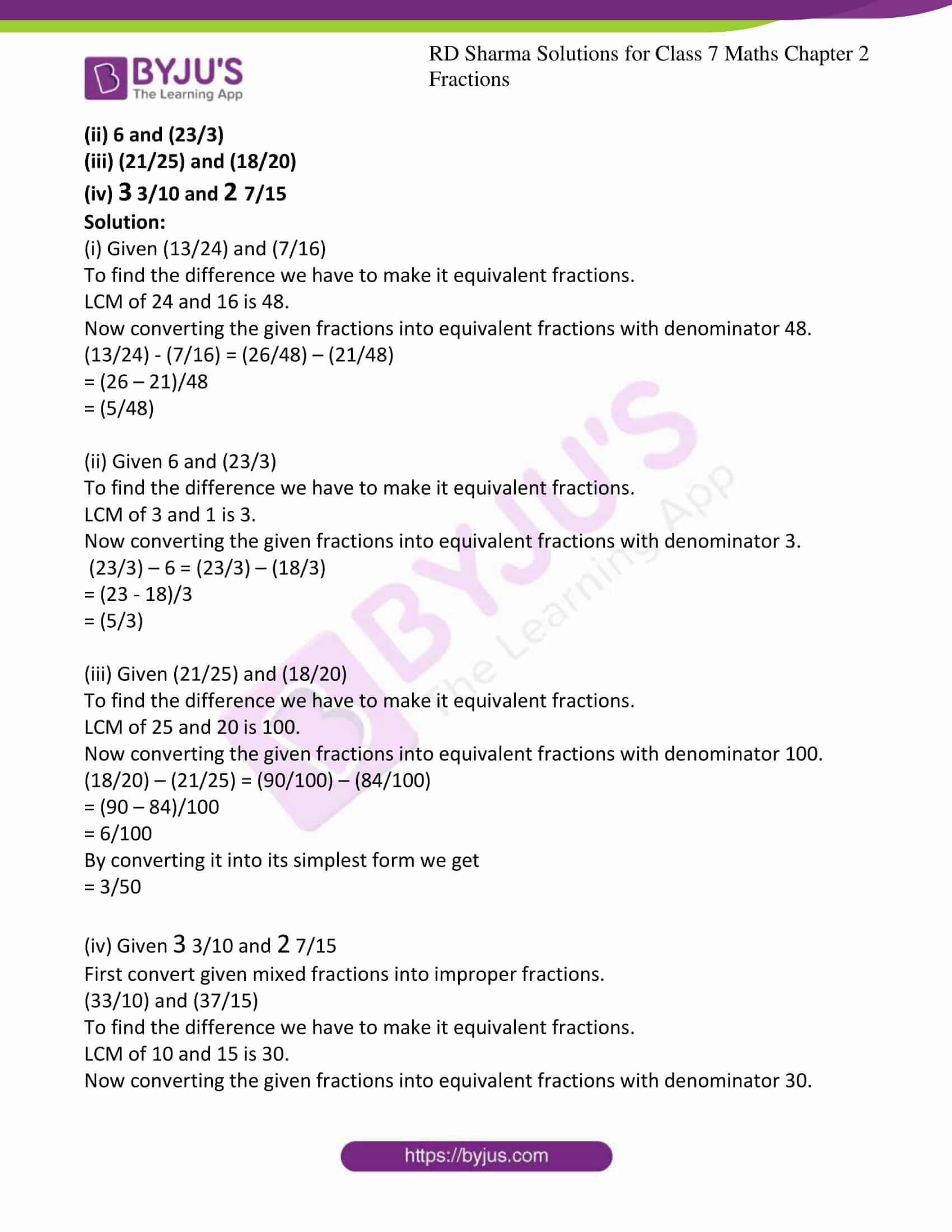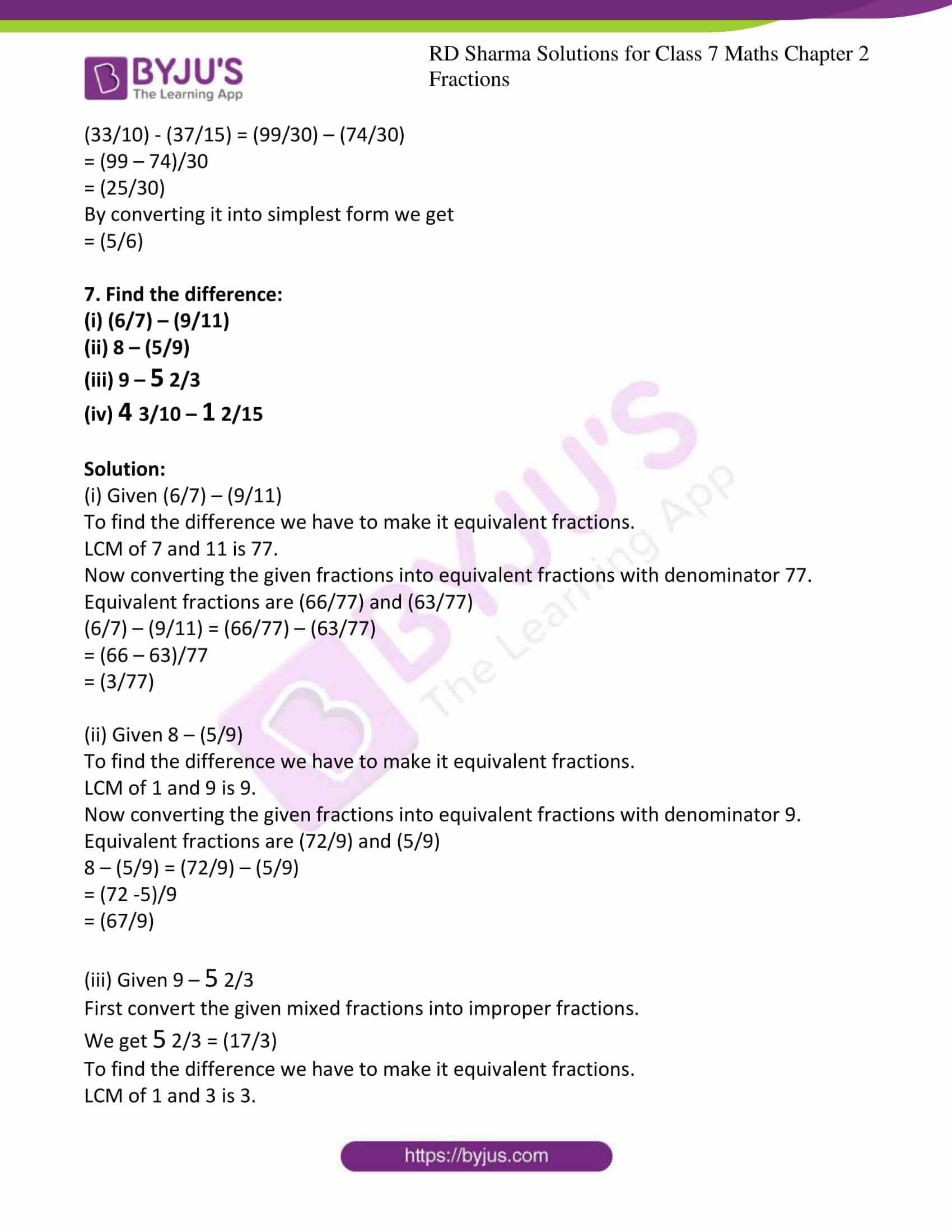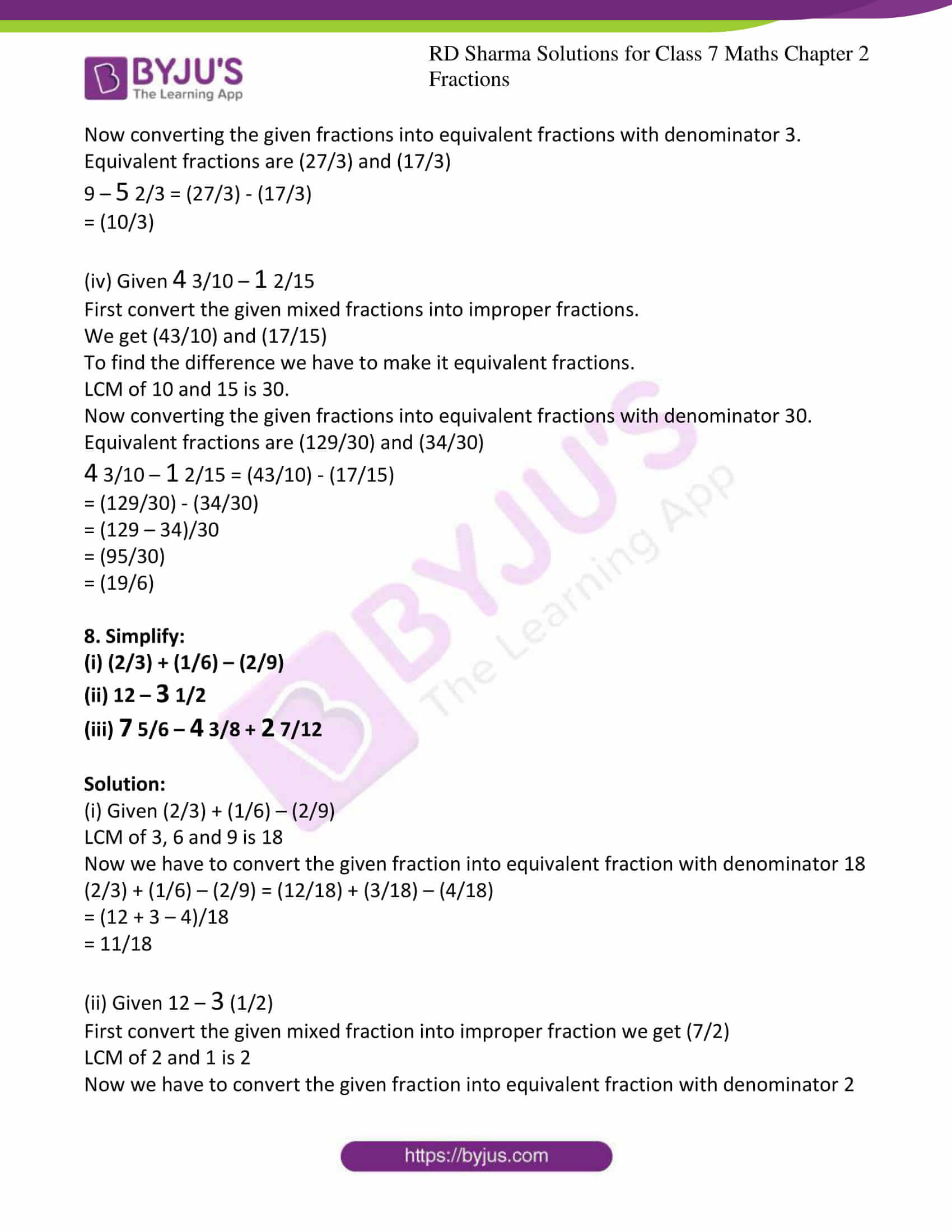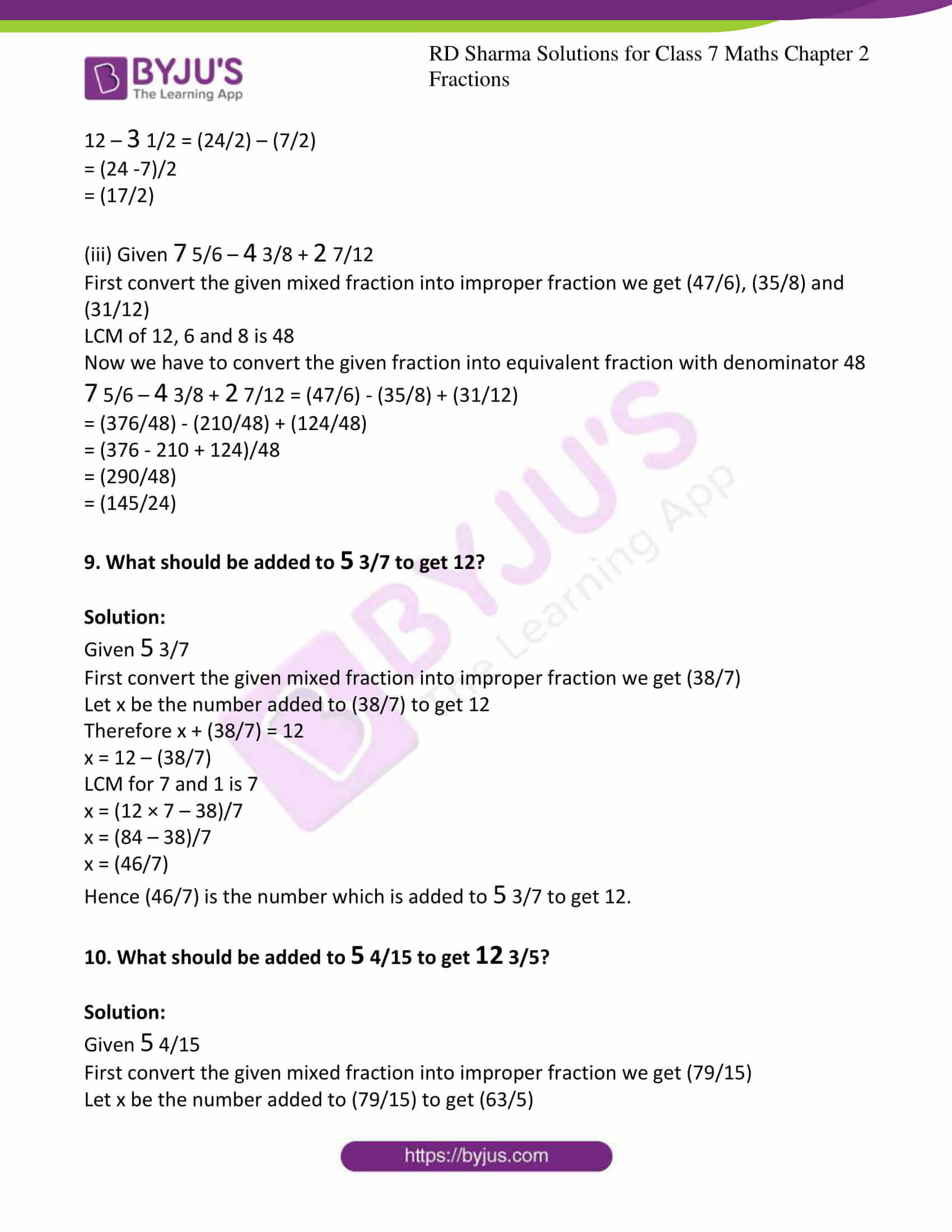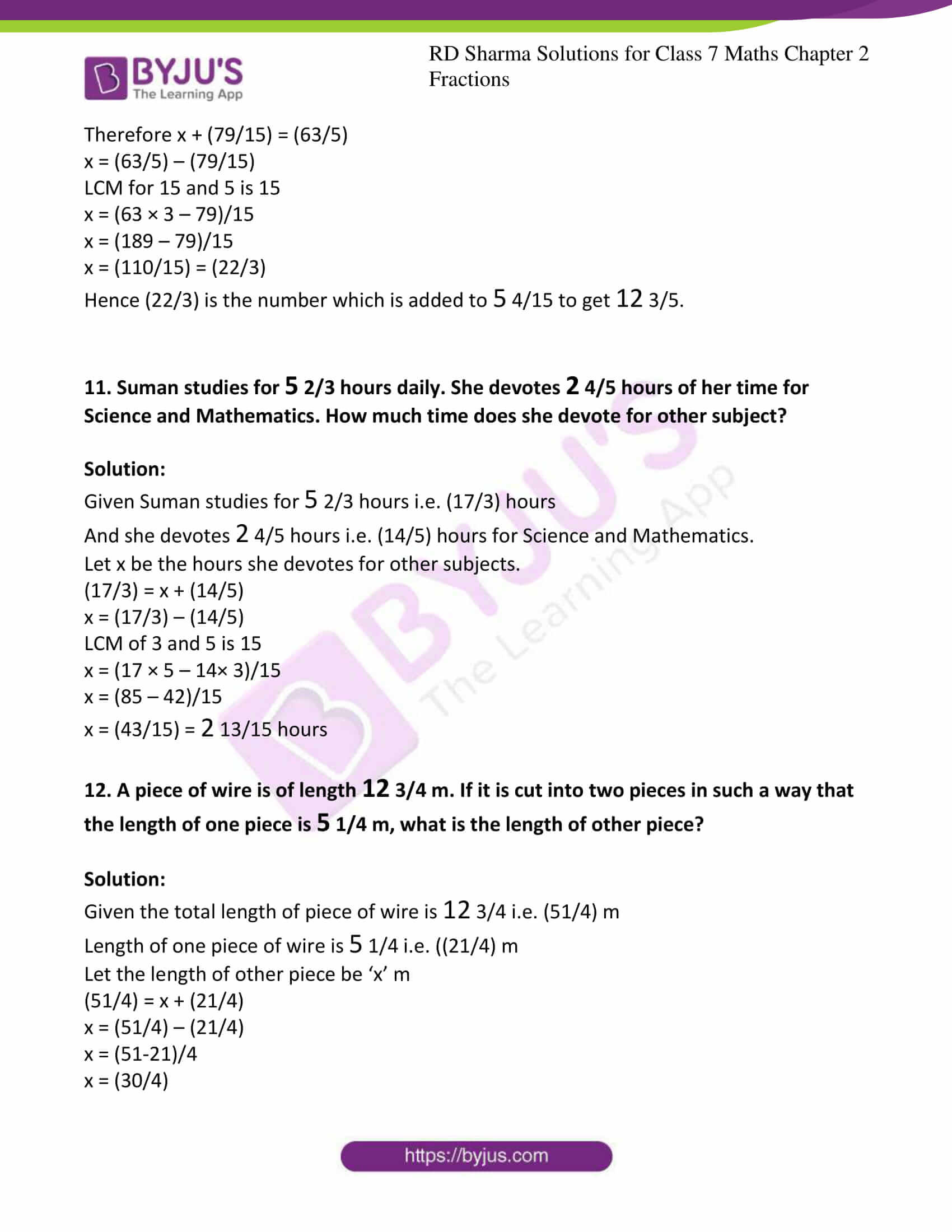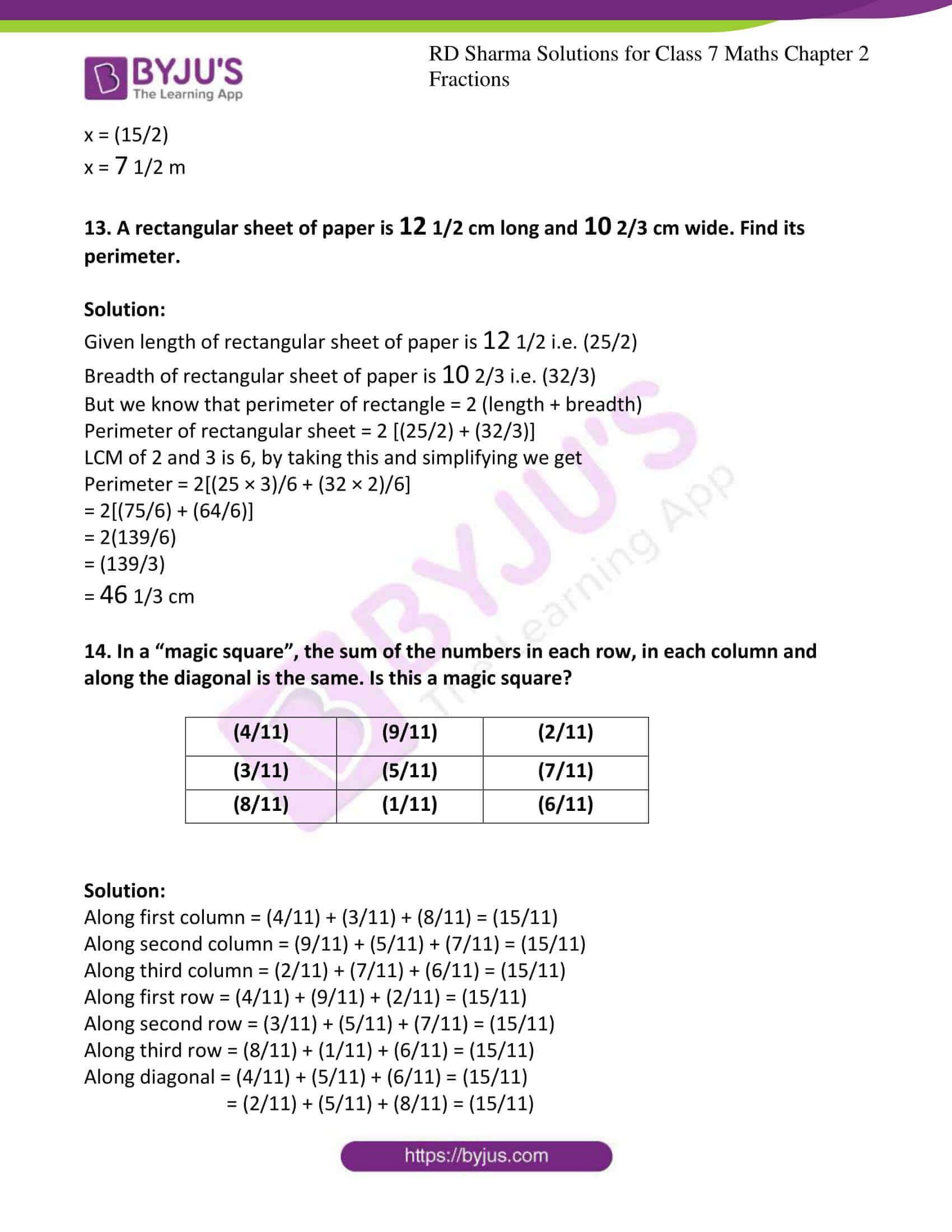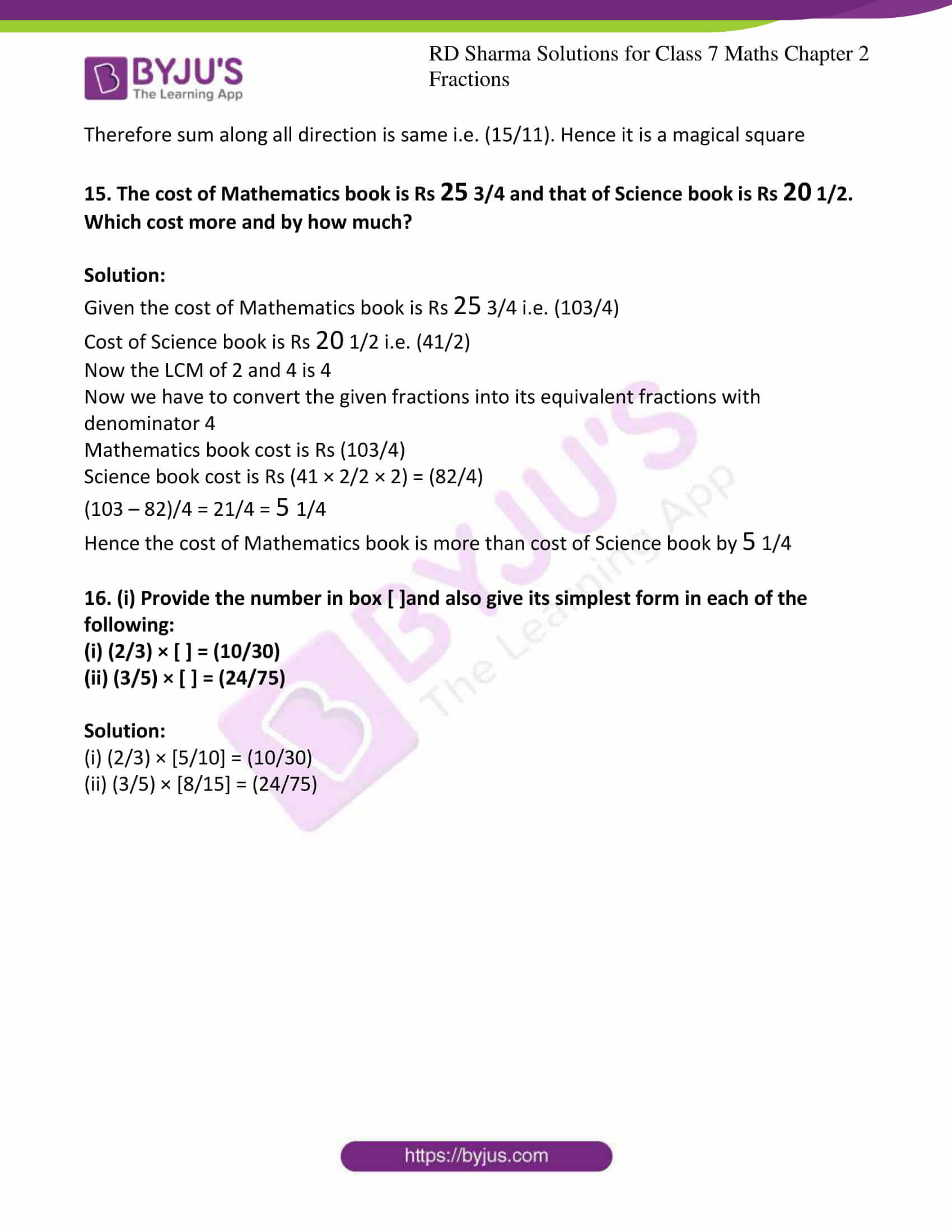### Access answers to Maths RD Sharma Solutions For Class 7 Chapter 2 – Fractions Exercise 2.1

Exercise 2.1 Page No: 2.12

1. Compare the following fractions by using the symbol > or < or =:

(i) (7/9) and (8/13)

(ii) (11/9) and (5/9)

(iii) (37/41) and (19/30)

(iv) (17/15) and (119/105)

Solution:

(i) Given (7/9) and (8/13)

Taking LCM for 9 and 13 we get,

9 × 13 = 117

Now we convert the given fractions into its equivalent fractions, then it becomes

(7 × 13)/ (9 × 13) and (8 × 9)/ (13 × 9)

Therefore (91/117) > (72/117)

Hence (7/9) > (8/13)

(ii) Given (11/9) and (5/9)

As the denominator is equal, they form equivalent fractions.

But we know that 11 > 5

Hence (11/9) > (5/9)

(iii) Given (37/41) and (19/30)

Taking LCM for 41 and 30 we get,

30 × 41 = 1230

Now we convert the given fractions into its equivalent fractions, then it becomes

(37 × 30)/ (41 × 30) and (19 × 41)/ (30 × 41)

Therefore (1110/1230) > (779/1230)

Hence (37/41) > (19/30)

(iv) Given (17/15) and (119/105)

Taking LCM for 15 and 105 we get, 5 × 3 × 7 = 105

Now we convert the given fractions into its equivalent fractions, then it becomes

(17 × 7)/ (15 × 7) and (119/105)

Therefore (119/105) = (119/105)

Hence (17/15) = (119/105)

2. Arrange the following fractions in ascending order:

(i) (3/8), (5/6), (6/8), (2/4), (1/3)

(ii) (4/6), (3/8), (6/12), (5/16)

Solution:

(i) Given (3/8), (5/6), (6/8), (2/4), (1/3)

Now we have to arrange these in ascending order, to arrange these in ascending order we have to make those as equivalent fractions by taking LCM’s.

LCM of 8, 6, 4 and 3 is 24

Equivalent fractions are

(9/24), (20/24), (18/24), (12/24), (8/24)

We know that 8 < 9 < 12 < 18 < 20

Now arranging in ascending order

(8/24) < (9/24) < (12/24) < (18/24) < (20/24)

Hence (1/3) < (3/8) < (2/4) < (6/8) < (5/6)

(ii) Given (4/6), (3/8), (6/12), (5/16)

Now we have to arrange these in ascending order, to arrange these in ascending order we have to make those as equivalent fractions by taking LCM’s.

LCM of 8, 6, 12 and 16 is 48

Equivalent fractions are

(32/48), (15/48), (18/48), (15/48)

We know that 12 < 15 < 18 < 32

Now arranging in ascending order

(12/48) < (15/48) < (18/48) < (32/48)

(6/12) < (5/16) < (3/8) < (4/6)

3. Arrange the following fractions in descending order:

(i) (4/5), (7/10), (11/15), (17/20)

(ii) (2/7), (11/35), (9/14), (13/28)

Solution:

(i) Given (4/5), (7/10), (11/15), (17/20)

Now we have to arrange these in descending order, to arrange these in descending order we have to make those as equivalent fractions by taking LCM’s.

LCM of 5, 10, 15 and 20 is 60

Equivalent fractions are

(48/60), (42/60), (44/60), (51/60)

We know that 51 > 48 > 44 > 42

Now arranging in descending order

Hence (17/20) > (4/5) > (11/15) > (7/10)

(ii) Given (2/7), (11/35), (9/14), (13/28)

Now we have to arrange these in descending order, to arrange these in descending order we have to make those as equivalent fractions by taking LCM’s.

LCM of 7, 35, 14 and 28 is 140

Equivalent fractions are

(40/140), (44/140), (90/140), (65/140)

We know that 90 > 65 > 44 > 40

Now arranging in descending order

Hence (9/14) > (13/28) > (11/35) > (2/7)

4. Write five equivalent fraction of (3/5).

Solution:

Given (3/5)

By multiplying or dividing both the numerator and denominator so that it keeps the same value by this we can get the equivalent fractions.

(3 × 2)/ (5 × 2), (3 × 3)/ (5 × 3), (3 × 4)/ (5 × 4), (3 × 5)/ (5 × 5), (3 × 6)/ (5 × 6)

Equivalent fractions are

(6/10), (9/15), (12/20), (15/25), (18/30)

5. Find the sum:

(i) (5/8) + (3/10)

(ii) 4 3/4 + 9 2/5

(iii) (5/6) + 3 + (3/4)

(iv) 2 3/5 + 4 7/10 + 2 4/15

Solution:

(i) Given (5/8) + (3/10)

Taking LCM for 8 and 10 we get 40

Now we have to convert the given fractions into equivalent fractions with denominator 40

(5/8) + (3/10) = (5 × 5)/ (8 × 5) + (3 × 4)/ (10 × 4)

= (25/40) + (12/40)

= (37/40)

(ii) Given 4 3/4 + 9 2/5

First convert given mixed fractions into improper fractions.

4 3/4 + 9 2/5 = (19/4) + (47/5)

Taking LCM for 4 and 5 we get 20

Now we have to convert the given fractions into equivalent fractions with denominator 20

4 3/4 + 9 2/5 = (19/4) + (47/5) = (19 × 5)/ (4 × 5) + (47 × 4)/ (5 × 4)

= (95/20) + (188/20)

= (283/20)

(iii) Given (5/6) + 3 + (3/4)

Taking LCM for 6, 1 and 4 we get 12

Now we have to convert the given fractions into equivalent fractions with denominator 12

(5/6) + 3 + (3/4) = (5 × 2)/ (6 × 2) + (3 × 12)/ (1 × 12) + (3 × 3)/ (4 × 3)

= (10/12) + (36/12) + (9/12)

= (55/12)

(iv) Given 2 3/5 + 4 7/10 + 2 4/15

First convert given mixed fractions into improper fractions

2 3/5 + 4 7/10 + 2 4/15 = (13/5) + (47/10) + (34/15)

Taking LCM for 5, 10 and 15 we get 30

Now we have to convert the given fractions into equivalent fractions with denominator 30

2 3/5 + 4 7/10 + 2 4/15 = (13/5) + (47/10) + (34/15) = (13 × 6)/ (5 × 6) + (47 × 3)/ (10 × 3) + (34 × 2)/ (15 × 2)

= (78/30) + (141/30) + (68/30)

= (287/30)

6. Find the difference of:

(i) (13/24) and (7/16)

(ii) 6 and (23/3)

(iii) (21/25) and (18/20)

(iv) 3 3/10 and 2 7/15

Solution:

(i) Given (13/24) and (7/16)

To find the difference we have to make it equivalent fractions.

LCM of 24 and 16 is 48.

Now converting the given fractions into equivalent fractions with denominator 48.

(13/24) – (7/16) = (26/48) – (21/48)

= (26 – 21)/48

= (5/48)

(ii) Given 6 and (23/3)

To find the difference we have to make it equivalent fractions.

LCM of 3 and 1 is 3.

Now converting the given fractions into equivalent fractions with denominator 3.

(23/3) – 6 = (23/3) – (18/3)

= (23 – 18)/3

= (5/3)

(iii) Given (21/25) and (18/20)

To find the difference we have to make it equivalent fractions.

LCM of 25 and 20 is 100.

Now converting the given fractions into equivalent fractions with denominator 100.

(18/20) – (21/25) = (90/100) – (84/100)

= (90 – 84)/100

= 6/100

By converting it into its simplest form we get

= 3/50

(iv) Given 3 3/10 and 2 7/15

First convert given mixed fractions into improper fractions.

(33/10) and (37/15)

To find the difference we have to make it equivalent fractions.

LCM of 10 and 15 is 30.

Now converting the given fractions into equivalent fractions with denominator 30.

(33/10) – (37/15) = (99/30) – (74/30)

= (99 – 74)/30

= (25/30)

By converting it into simplest form we get

= (5/6)

7. Find the difference:

(i) (6/7) – (9/11)

(ii) 8 – (5/9)

(iii) 9 – 5 2/3

(iv) 4 3/10 – 1 2/15

Solution:

(i) Given (6/7) – (9/11)

To find the difference we have to make it equivalent fractions.

LCM of 7 and 11 is 77.

Now converting the given fractions into equivalent fractions with denominator 77.

Equivalent fractions are (66/77) and (63/77)

(6/7) – (9/11) = (66/77) – (63/77)

= (66 – 63)/77

= (3/77)

(ii) Given 8 – (5/9)

To find the difference we have to make it equivalent fractions.

LCM of 1 and 9 is 9.

Now converting the given fractions into equivalent fractions with denominator 9.

Equivalent fractions are (72/9) and (5/9)

8 – (5/9) = (72/9) – (5/9)

= (72 -5)/9

= (67/9)

(iii) Given 9 – 5 2/3

First convert the given mixed fractions into improper fractions.

We get 5 2/3 = (17/3)

To find the difference we have to make it equivalent fractions.

LCM of 1 and 3 is 3.

Now converting the given fractions into equivalent fractions with denominator 3.

Equivalent fractions are (27/3) and (17/3)

9 – 5 2/3 = (27/3) – (17/3)

= (10/3)

(iv) Given 4 3/10 – 1 2/15

First convert the given mixed fractions into improper fractions.

We get (43/10) and (17/15)

To find the difference we have to make it equivalent fractions.

LCM of 10 and 15 is 30.

Now converting the given fractions into equivalent fractions with denominator 30.

Equivalent fractions are (129/30) and (34/30)

4 3/10 – 1 2/15 = (43/10) – (17/15)

= (129/30) – (34/30)

= (129 – 34)/30

= (95/30)

= (19/6)

8. Simplify:

(i) (2/3) + (1/6) – (2/9)

(ii) 12 – 3 1/2

(iii) 7 5/6 – 4 3/8 + 2 7/12

Solution:

(i) Given (2/3) + (1/6) – (2/9)

LCM of 3, 6 and 9 is 18

Now we have to convert the given fraction into equivalent fraction with denominator 18

(2/3) + (1/6) – (2/9) = (12/18) + (3/18) – (4/18)

= (12 + 3 – 4)/18

= 11/18

(ii) Given 12 – 3 (1/2)

First convert the given mixed fraction into improper fraction we get (7/2)

LCM of 2 and 1 is 2

Now we have to convert the given fraction into equivalent fraction with denominator 2

12 – 3 1/2 = (24/2) – (7/2)

= (24 -7)/2

= (17/2)

(iii) Given 7 5/6 – 4 3/8 + 2 7/12

First convert the given mixed fraction into improper fraction we get (47/6), (35/8) and (31/12)

LCM of 12, 6 and 8 is 48

Now we have to convert the given fraction into equivalent fraction with denominator 48

7 5/6 – 4 3/8 + 2 7/12 = (47/6) – (35/8) + (31/12)

= (376/48) – (210/48) + (124/48)

= (376 – 210 + 124)/48

= (290/48)

= (145/24)

9. What should be added to 5 3/7 to get 12?

Solution:

Given 5 3/7

First convert the given mixed fraction into improper fraction we get (38/7)

Let x be the number added to (38/7) to get 12

Therefore x + (38/7) = 12

x = 12 – (38/7)

LCM for 7 and 1 is 7

x = (12 × 7 – 38)/7

x = (84 – 38)/7

x = (46/7)

Hence (46/7) is the number which is added to 5 3/7 to get 12.

10. What should be added to 5 4/15 to get 12 3/5?

Solution:

Given 5 4/15

First convert the given mixed fraction into improper fraction we get (79/15)

Let x be the number added to (79/15) to get (63/5)

Therefore x + (79/15) = (63/5)

x = (63/5) – (79/15)

LCM for 15 and 5 is 15

x = (63 × 3 – 79)/15

x = (189 – 79)/15

x = (110/15) = (22/3)

Hence (22/3) is the number which is added to 5 4/15 to get 12 3/5.

11. Suman studies for 5 2/3 hours daily. She devotes 2 4/5 hours of her time for Science and Mathematics. How much time does she devote for other subject?

Solution:

Given Suman studies for 5 2/3 hours i.e. (17/3) hours

And she devotes 2 4/5 hours i.e. (14/5) hours for Science and Mathematics.

Let x be the hours she devotes for other subjects.

(17/3) = x + (14/5)

x = (17/3) – (14/5)

LCM of 3 and 5 is 15

x = (17 × 5 – 14× 3)/15

x = (85 – 42)/15

x = (43/15) = 2 13/15 hours

12. A piece of wire is of length 12 3/4 m. If it is cut into two pieces in such a way that the length of one piece is 5 1/4 m, what is the length of other piece?

Solution:

Given the total length of piece of wire is 12 3/4 i.e. (51/4) m

Length of one piece of wire is 5 1/4 i.e. ((21/4) m

Let the length of other piece be ‘x’ m

(51/4) = x + (21/4)

x = (51/4) – (21/4)

x = (51-21)/4

x = (30/4)

x = (15/2)

x = 7 1/2 m

13. A rectangular sheet of paper is 12 1/2 cm long and 10 2/3 cm wide. Find its perimeter.

Solution:

Given length of rectangular sheet of paper is 12 1/2 i.e. (25/2)

Breadth of rectangular sheet of paper is 10 2/3 i.e. (32/3)

But we know that perimeter of rectangle = 2 (length + breadth)

Perimeter of rectangular sheet = 2 [(25/2) + (32/3)]

LCM of 2 and 3 is 6, by taking this and simplifying we get

Perimeter = 2[(25 × 3)/6 + (32 × 2)/6]

= 2[(75/6) + (64/6)]

= 2(139/6)

= (139/3)

= 46 1/3 cm

14. In a “magic square”, the sum of the numbers in each row, in each column and along the diagonal is the same. Is this a magic square?

 (4/11) (9/11) (2/11) (3/11) (5/11) (7/11) (8/11) (1/11) (6/11)

Solution:

Along first column = (4/11) + (3/11) + (8/11) = (15/11)

Along second column = (9/11) + (5/11) + (7/11) = (15/11)

Along third column = (2/11) + (7/11) + (6/11) = (15/11)

Along first row = (4/11) + (9/11) + (2/11) = (15/11)

Along second row = (3/11) + (5/11) + (7/11) = (15/11)

Along third row = (8/11) + (1/11) + (6/11) = (15/11)

Along diagonal = (4/11) + (5/11) + (6/11) = (15/11)

= (2/11) + (5/11) + (8/11) = (15/11)

Therefore sum along all direction is same i.e. (15/11). Hence it is a magical square

15. The cost of Mathematics book is Rs 25 3/4 and that of Science book is Rs 20 1/2. Which cost more and by how much?

Solution:

Given the cost of Mathematics book is Rs 25 3/4 i.e. (103/4)

Cost of Science book is Rs 20 1/2 i.e. (41/2)

Now the LCM of 2 and 4 is 4

Now we have to convert the given fractions into its equivalent fractions with denominator 4

Mathematics book cost is Rs (103/4)

Science book cost is Rs (41 × 2/2 × 2) = (82/4)

(103 – 82)/4 = 21/4 = 5 1/4

Hence the cost of Mathematics book is more than cost of Science book by 5 1/4

16. (i) Provide the number in box [ ]and also give its simplest form in each of the following:

(i) (2/3) × [ ] = (10/30)

(ii) (3/5) × [ ] = (24/75)

Solution:

(i) (2/3) × [5/10] = (10/30)

(ii) (3/5) × [8/15] = (24/75)

## RD Sharma Solutions for Class 7 Maths Chapter – 2 Fractions Exercise 2.1

Exercise 2.1 of RD Sharma Solutions for Chapter 2, Fractions explains the definition of fractions and fundamental operations on them. Some of the topics focused prior to Exercise 2.1 are listed below.

• Definition of fractions
• Types of fractions
• Fraction in lowest terms
• Comparing fractions
• Conversion of unlike fractions to like fractions